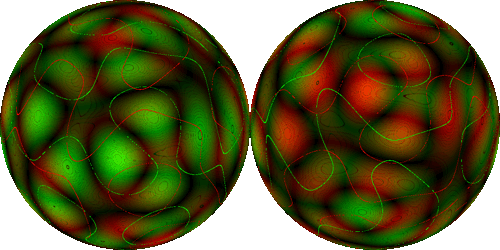Antipodal displays the contours of two continuous functions on the surface of a sphere, as well as curves (red and green) showing the points where the value of each function is equal to that at the antipodal point.

Click on the applet to pause or redraw.

Every great circle on the sphere must contain at least one pair of antipodal points where the value of either function is equal. To see this, suppose the function is F, and we choose an angular coordinate φ that varies from 0 to 2π around the great circle. We define:

D(φ) = F(φ)–F(φ+π)

So D is the difference in F between one point on the great circle and the antipodal point. By definition, D(0) = –D(π), so either D(0)=D(π)=0, or D(φ) changes sign between 0 and π, so by the Intermediate Value Theorem D(φ) must be zero for some 0 < φ < π.

Furthermore, there must be at least one pair of antipodal points on the sphere where both functions take the same value. This is the Borsuk-Ulam Theorem. In the applet, these points can be seen where the red and green curves intersect. In general, the Borsuk-Ulam Theorem says that any map from the n-dimensional sphere to Rn must take at least one pair of antipodal point on the n-sphere to the same n-tuple in Rn.Applets Gallery / Antipodal / created Saturday, 11 August 2018